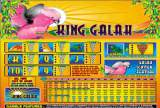# King Galah

## Slot Machine published 24 years ago by Aristocrat Leisure Industries Pty

#### Listed and emulated in MAME.

A 5-reel video slot machine with an Australian outback theme.Click to enlarge
(members only)
TECHNICAL

Aristocrat MK5 hardware.

Video Resolution : 640x400
Colours: 256
CPU : ARM250
Controls : 14 buttons

Button layout, 200 credit version:

Top row buttons :
Collect
Bet 1 Credit / Heart
Bet 2 Credits / Diamond
Bet 3 Credits
Bet 5 Credits / Club

Bottom row buttons :
Service
Play 1 Line / Red
Play 5 Lines
Play 10 Lines
Play 15 Lines
Play 20 Lines / Black
Gamble
Take Win

SCORING

All pays are left to right including scatters.
Lizard substitutes for all symbols except Galahs.

Lizard : 5 = 5000, 4 = 1000, 3 = 500, 2 = 20, 1 = 2
Ayers Rock : 5 = 1000, 4 = 500, 3 = 100, 2 = 10
Dingo : 5 = 500, 4 = 100, 3 = 20, 2 = 5
Gum Leaf : 5 = 500, 4 = 100, 3 = 20, 2 = 5
King : 5 = 200, 4 = 50, 3 = 20
Queen : 5 = 200, 4 = 50, 3 = 20
Jack : 5 = 100, 4 = 20, 3 = 10
Ten : 5 = 100, 4 = 20, 3 = 5
Nine : 5 = 100, 4 = 20, 3 = 5, 2 = 2
Galah : Varies (see Tips & Tricks below)

TIPS AND TRICKS

* Galah Scatter Feature : Scattered Galahs pay depending on their position. Scatter wins are multiplied by total bet (credit x lines) on that spin.

Per 1 credit bet :
In centre : 5 = 500, 4 = 100, 3 = 50
On and above/below the centre : 5 = 100, 4 = 20, 3 = 10
Anywhere in window : 5 = 50, 4 = 10, 3 = 5

SERIES

1) King Galah (1997)
2) Macaw Magic (2006)

SOURCES

Game's ROMs.